# Delta Hausdorff Dimension

Robert P. Munafo, 2010 Sep 7.

The box-counting dimension for a given neighborhood of a point, which is considered to be a term in a series, the limit of which would be the Hausdorff dimension.

It gives a rough qualitative estimate of the "apparent" Hausdorff dimension of an image at a given pixel resolution, and is computed by the following formula:

Ddh = log2(POP2s / POPs)

Here Ddh represents delta Hausdorff dimension, POP2s is the population (pixel count) on a pixel grid of size 2s, and POPs is the population on a grid of size s. Both pixel grids cover the same area (as expressed in real and imaginary coordinates) but the second grid has twice as many pixels (sample-points).

If P is a point in the Mandelbrot Set, Ddh for a neighborhood of P is constrained to be in the range containing the Hausdorff dimensions of the Julia Sets for all points within the neighborhood. There are points in the Mandelbrot Set for which the Julia Set has a Hausdorff dimension "arbitrarily close" to 2.0. Since all neighborhoods of P contain embedded copies of the Mandelbrot Set, the upper bound of the range for any neighborhood is 2.0. As you zoom in, the neighborhood gets smaller and the lower bound of the range can go up. However the lower bound does not always go up. Also, the Ddh of the neighborhood can go up or down as you zoom in.

This is all sort of abstract, so I'll give some examples. To appreciate what's going on, compare the images to the numbers below. The number gives the fractal dimension of the image by the box-counting method; it can be anything from 1.00 to 2.00. The images were created using the distance estimator method.

In the first example, we're zooming in to the tip of a filament and Ddh goes down and reaches an asymptote quickly:

 coordinates 0.00000 +1.00000i radius 0.3Ddh = 1.540 radius 0.1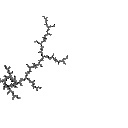Ddh = 1.349 radius 0.03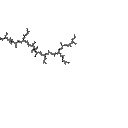Ddh = 1.255 radius 0.01Ddh = 1.238 radius 0.003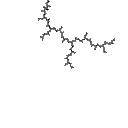Ddh = 1.278 radius 0.001Ddh = 1.248 radius 0.0003Ddh = 1.278 radius 0.0001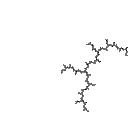Ddh = 1.257

In the second example Ddh goes up steadily as we zoom in, which is the most common behavior for most points that people usually zoom in to:

 coordinates -1.74856 +0.00075i radius 0.3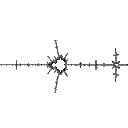Ddh = 1.275 radius 0.1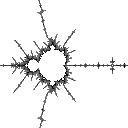Ddh = 1.389 radius 0.03Ddh = 1.547 radius 0.01Ddh = 1.548 radius 0.003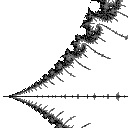Ddh = 1.594 radius 0.001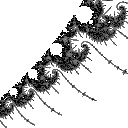Ddh = 1.663 radius 0.0003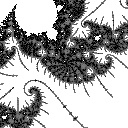Ddh = 1.706 radius 0.0001Ddh = 1.765

In the third example Ddh goes up and then goes back down:

 coordinates -1.74831 +0.00046i radius 0.3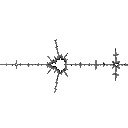Ddh = 1.361 radius 0.1Ddh = 1.400 radius 0.03Ddh = 1.568 radius 0.01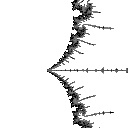Ddh = 1.602 radius 0.003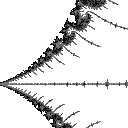Ddh = 1.583 radius 0.001Ddh = 1.602 radius 0.0003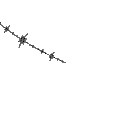Ddh = 1.357 radius 0.0001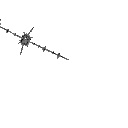Ddh = 1.382

It can occur that Ddh goes up and down several or many times, but you have to be willing to zoom in a really long way to see this happen. One example is the point

-1.769 110 375 463 767 385 + 0.009 020 388 228 023 440 i

if you zoom in "all the way" on these coordinates you see this:the Munafo midget

References

This page was written in the "embarrassingly readable" markup language RHTF, and was last updated on 2020 Mar 26.s.11